## Journal of Physics Research and Applications

All submissions of the EM system will be redirected to Online Manuscript Submission System. Authors are requested to submit articles directly to Online Manuscript Submission System of respective journal.

Editorial, J Phys Res Appl Vol: 1 Issue: 1

# Finding a Method for Producing Small Impedance Particles with Prescribed Boundary Impedance is Important

Ramm AG*

Department of Mathematics, Kansas State University, Manhattan, USA

*Corresponding Author : Alexander G Ramm
Department of Mathematics, Kansas State University, Manhattan, USA
Tel: (785) 532-0580
E-mail: ramm@math.ksu.edu

Received: November 10, 2017 Accepted: November 13, 2017 Published: November 17, 2017

Citation: Ramm AG (2017) Finding a Method for Producing Small Impedance Particles with Prescribed Boundary Impedance is Important. J Phys Res Appl 1:1.

## Abstract

The problem of practical preparing small impedance particles with prescribed boundary impedance is formulated and its importance in physics and technology is emphasized. If this problem is solved then materials with a desired refraction coefficient can be prepared. These include meta-materials and materials with a desired radiation pattern. Some arguments are given to prove the existence of small particles with the prescribed boundary impedance.

## Introduction

Creating materials with a desired refraction coefficient is a problem of major scientific and technological importance. These materials include meta materials, that is, materials in which group velocity is directed opposite to the phase velocity; materials with a desired radiation pattern, that is materials with a desired scattering amplitudes at a fixed frequency and a fixed incident wave direction. Such materials may scatter the incident plane wave so that most of the energy of the scattered wave goes, for example, in a desired cone. The author developed a theory of wave scattering by many small impedance particles with prescribed boundary impedance [1,10]. This theory allows one to give a recipe for creating materials with a desired refraction coefficient by distributing (with some distribution density) in a given material many small impedance particles with a prescribed boundary impedance. The recipe is formulated in this paper. In order to use it practically one has to solve two technological problems:

1. How to distribute (embed) in a given material many small impedance particles,

and

2. How to produce a small particle with prescribed boundary impedance.

The first of these problems is currently solved.

The aim of this paper is to draw attention of physicists, materials science specialists and engineers to solving the second technological problem. The contents of this paper is very close to the paper of Ramm AG , not yet published, and parts of this paper are taken from  verbatim. Wave scattering by many small impedance particles is developed in the book of Ramm AG , where the basic physical assumption is É « d « λ. Here ‘É’ is the characteristic size of the small particles, ‘d’ is the minimal distance between neighboring particles, and λ is the wave length in the medium. It is proved there that if one prepares many small particles with prescribed boundary impedance and embeds these particles (with a specified in  distribution density, see formula (7) below) into a given material, then one obtains a material whose refraction coefficient approximates any desired refraction coefficient with an arbitrary small error.

Therefore the basic practical problem of preparing small impedance particles with prescribed boundary impedance is of great interest both technologically and physically.

In section 2 the basic definitions are given. It is explained what a small particle is, what an impedance particle is, what a boundary impedance is. We argue that impedance boundary condition makes physical sense on a particle of arbitrary size and that a small particle with prescribed boundary impedance should exist.

The problem is: how can one produce (manufacture) such a particle practically?

In section 3 a recipe for creating materials with a desired refraction coefficient is formulated. This recipe is taken from the author’s monograph of Ramm AG .

## Basic Definitions

Let D1⊂ R3 be a bounded domain with a connected smooth boundary S1, D′1 :=R3 \ D′1 be the unbounded exterior domain and S2 be the unit sphere in R3.

Consider the scattering problem:(1)

where k > 0 is the wave number, a constant, α ∈ S2 is a unit vector in the direction of the propagation of the incident plane wave eikα•x, N is the unit normal to S1 pointing out of D1, uN is the normal derivative of u, ζ1 is the boundary impedance, Imζ1 ≤ 0, n0 > 0 is the refraction coefficient of the small impedance particle D1, n0 is a constant, the scattered field v satisfies the radiation condition(2)

The scattering amplitude A(β,α, k) is defined by the following formula:(3)

where α, β ∈ S2, β is the direction of the scattered wave, α is the direction of the incident wave. A particle of a characteristic size a is called small if it is much smaller than the wave length in the medium, that is, kn0É « 1.

The function A(β, α, k) is called the scattering amplitude. It is known (see Ramm AG , p.25, or , ) that the solution to the scattering problem (1)-(3) does exist and is unique.

If there are many (M » 1) small impedance particles Dj, embedded in a bounded domain D filled with material whose refraction coefficient is n0, then the wave scattering problem can be formulated as follows:(4)(5)(6)

where the scattered field v satisfies the radiation condition, Imζm ≤ 0, M is the total number of the embedded particles. The incident field u0 is assumed known. It satisfies equation (4) in R3, and, as was noted earlier, n0 = 1 in D’. For simplicity we assume here that all small particles are of the same characteristic size É. In Ramm AG  small particles of various sizes and shapes are allowed.

Let G be the Green’s function of the scattering problem in the absence of the embedded particles. Outside D the refraction coefficient n0 is assumed to be equal to 1. Assume that the distribution of small particles is given by the formula(7)

where(Δ) is the number of particles in an arbitrary open subset of D, N (x) ≥ 0 is a given continuous function, κ ∈ [0, 1) is a number, and the boundary impedance is defined as follows:(8)

where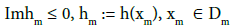is an arbitrary point and h(x) is a given continuous function in D. This function, number κ and the function N (x) can be chosen by the experimenter. It is proved in Ramm AG . p. 48. that the field u in D satisfies, as

É → 0, the following integral equation: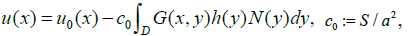(9)

where S is the surface area of a small particle, h(x) and N(x) are defined in (7)-(8). For simplicity we assume here that the surface area S is the same for all small particles. It follows from (9) that the new refraction coefficient in D, which one gets after embedding many small impedance particles, is(10)

Since h(x) and N (x) are at our disposal, one can get by formula (10) any desirable refraction coefficient, such that Imn2(x) ≥ 0.

Why should the equation (5) make sense physically regardless of the size of the particle?

Because a problem whose solution exists and is unique must have sense physically.

Why should the small impedance particles with prescribed boundary impedance exist?

Because the particles with ζ=∞, acoustically soft particles, do exist, and the particles with ζ=0, acoustically hard particles, do exist, we conclude that small particles with any “intermediate” value of the boundary impedance should also exist.

The problem we raise is:

How can one produce practically (fabricate) such particles?

## Recipe for Creating Material with a Desired Refraction Coefficient

Let us formulate the result in the following theorem

Theorem 1. Given n2(x) and a bounded domain D, one can create in D a material with a desired refraction coefficient n(x), Imn2(x) ≥ 0, by embeddingsmall impedance particles according to the distribution law (7). The refraction coefficientcorresponding to a finite É, approximates the desired refraction coefficient n(x) in the sense(11)

The functions h(x) and N (x) defined in (7)-(8) are found by the Steps 1, 2 of the Recipe formulated below; see also p. 53 in .

Finally, let us discuss briefly the possibility to create material with negative refraction coefficient.

By formulas (3.1.1) and (3.1.2) in  one gets n(x) < 0 if the argument ofis equal to 2π. Assume thatWe know thatLet us take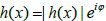where Ï > 0 is very small, that is, Imh(x) ≥ 0 and Imh(x) is very small. One proves (see also , p.52) that equation (9) is uniquely solvable for Imh ≥ 0 sufficiently small. For such h(x) one concludes that the argumentis very close to 2π and the square root in (10) is negative, provided that. This argument shows that it is possible to create materials with negative refraction coefficient n(x) by embedding in a given material many small particles with properly chosen boundary impedances (6).

A recipe for creating materials with a desired refraction coefficient

Problem 1. Given a material with a known n0(x) in a bounded domain D and n0(x) = 1 in D′ one wants to create in D a material with a desired n(x).

Step 1. Givenand n2(x), calculateThis is a trivial step.

Step 2. Given p(x), calculate h(x) and N (x) from the equation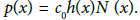The constant c0 one can take to be c = 4π if the small particles are balls of radius a. This we can assume without loss of generality if we are interested in creating materials with a desired refraction coefficient .

There are infinitely many solutions h and N to the above equation . For example, one can fix arbitrarily

N (x) > 0 in D, N (x) = 0 in D′ and find h(x) = h1(x) + ih2(x) by the formulas:Here p1(x)= Rep(x) and p2(x) = Imp(x).

Note that Imn2(x) ≥ 0 implies Imh(x) ≤ 0, so our assumption Imh(x) ≤ 0 is satisfied. For example, one may take N (x) = const > 0.

Step 2 is also trivial.

Step 3. Given N (x) and h(x) = h1(x) + ih2(x), distributesmall impedance balls of radius a in the bounded region D according to the distribution law (7), where κ ∈ [0, 1) is the number (parameter) that can be chosen by the experimenter. Note that condition d » a is satisfied automatically for the distribution law (7). Indeed, if d is the minimal distance between neighboring particles, then there are at most 1/d3 particles in a cube with the unit side, and since D is a bounded domain there are at most O(1/d3) of small particles in D. On the other hand, by the distribution law (7) one has,Thus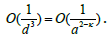Therefore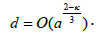Consequently, condition a « d « λ is satisfied, whereMoreover## References## Explore SciTechnol

###### Citations : 19

Journal of Physics Research and Applications received 19 citations as per Google Scholar report

## Recommended Conferences

### 9th International Conference on Physics

Edinburgh, Scotland

### 6th International Conference on Astronomy, Astrophysics and Space Science

Budapest, Hungary